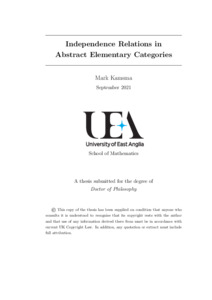# Independence relations in abstract elementary categories

Kamsma, Mark (2021) Independence relations in abstract elementary categories. Doctoral thesis, University of East Anglia.Preview PDF Download (1MB) | Preview

## Abstract

In model theory, a branch of mathematical logic, we can classify mathematical structures based on their logical complexity. This yields the so-called stability hierarchy. Independence relations play an important role in this stability hierarchy. An independence relation tells us which subsets of a structure contain information about each other, for example: linear independence in vector spaces yields such a relation.

Some important classes in the stability hierarchy are stable, simple and NSOP1, each being contained in the next. For each of these classes there exists a so-called Kim-Pillay style theorem. Such a theorem describes the interaction between independence relations and the stability hierarchy. For example: simplicity is equivalent to admitting a certain independence relation, which must then be unique.

All of the above classically takes place in full first-order logic. Parts of it have already been generalised to other frameworks, such as continuous logic, positive logic and even a very general category-theoretic framework. In this thesis we continue this work.

We introduce the framework of AECats, which are a specific kind of accessible category. We prove that there can be at most one stable, simple or NSOP1-like independence relation in an AECat. We thus recover (part of) the original stability hierarchy. For this we introduce the notions of long dividing, isi-dividing and long Kim-dividing, which are based on the classical notions of dividing and Kim-dividing but are such that they work well without compactness.

Switching frameworks, we generalise Kim-dividing in NSOP1 theories to positive logic. We prove that Kim-dividing over existentially closed models has all the nice properties that it is known to have in full first-order logic. We also provide a full Kim-Pillay style theorem: a positive theory is NSOP1 if and only if there is a nice enough independence relation, which then must be given by Kim-dividing.

Item Type: Thesis (Doctoral) Faculty of Science > School of Mathematics Chris White 15 Jun 2022 08:18 15 Jun 2022 08:18 https://ueaeprints.uea.ac.uk/id/eprint/85625View Item